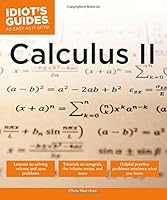# Calculus II (Idiot’s Guides)0 Reviews
2016-12-13
336 pages

## Book Description

Idiot's Guides: II, like its counterpart Idiot's Guides: Calculus I, is a curriculum-based companion book that continues the tradition of taking the sting out of calculus by adding more explanatory and illustrations in easy-to-understand language, practice problems, and even a test at the end. Idiot's Guides: Calculus II is geared for all students who need to succeed in calculus.

Also included:

• Advanced and complex problem examples.
• Sidebar problems sprinkled throughout to test reader's knowledge with answer key in the back.
• Practice test included at the end of the book, complete with answer key.

Part 1: Review of Pre-Calculus and Calculus I
Chapter 1 Pre-Calculus Topics Used In Calculus II
Chapter 2 Limits, Derivatives, And Basic
Chapter 3 Definite And Indefinite Integrals

Part 2: Length, Area, and Volumes
Chapter 4 Areas And Approximations
Chapter 5 Volumes And Areas Of Solids Of Revolutions

Part 3: More Definite and Indefinite Integrals
Chapter 6 More Integration Techniques
Chapter 7 Integration With Trigonometric
Chapter 8 Integration With Fractions

Part 4: The Infinite Series and More
Chapter 9 To Infinity And Beyond
Chapter 10 Parametric Equations
Chapter 11 Polar Coordinates
Chapter 12 Introduction To Vectors
Chapter 13 Differential Equations
Chapter 14 Infinite Sequences
Chapter 15 Infinite Series
Chapter 16 Power Series
Chapter 17 Calculus Ii Final Exam

Appendix A Solutions To “You’Ve Got Problems”
Appendix B Integration Practice Problems And Solutions
Appendix C Glossary

## Book Details

• Title: Calculus II (Idiot’s Guides)
• Author:
• Length: 336 pages
• Edition: 1
• Language: English
• Publisher:
• Publication Date: 2016-12-13
• ISBN-10: 1465454403
• ISBN-13: 9781465454409